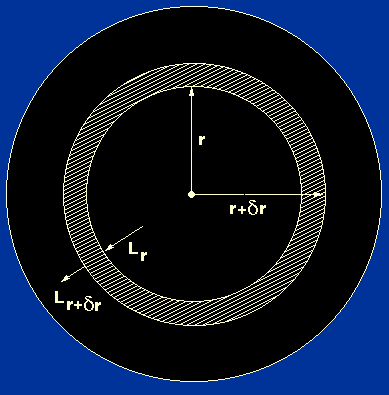Returning to the derivation of the equations of stellar structure, we can immediately write down one equation which relates the rate of energy release to the rate of energy transport.

Consider a spherically symmetric star in which energy transport is radial and in which time variations are unimportant. Lr is the rate of energy flow across a sphere of radius r and Lr+r is the rate of energy flow across a sphere of radius r +r, as shown in figure 9.

 Figure 9: A thin spherical shell inside a star.Because the shell is thin, its volume is given by its surface area times its thickness:

4r2r.

Recalling that mass = density x volume, the mass of the shell is then given by:

4r2r.

The energy released in the shell can then be written as

4r2r,

whereis defined as the energy release per unit mass per unit time (W kg-1).

The conservation of energy leads us to equate the energy crossing the sphere at r +r to the sum of the energy crossing the sphere at r and the energy released in the shell, as follows:

Lr+r = Lr + 4r2r,

For a thin shell we can also write:

Lr+r - Lr = (dL / dr)r            (in the limitr -> 0,L /r = dL / dr, whereL = Lr+r - Lr ).

Equating these last two equations gives:

dL / dr = 4r2.

We shall call this the equation of energy production.We now have three of the equations of stellar structure. This is not yet a complete set of equations as we have five unknowns (P, M,, L and). In order to make further progress, we need to consider energy transport in stars.